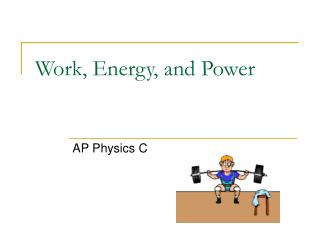# Work, Energy, and Power - PowerPoint PPT PresentationDownload PresentationWork, Energy, and Power

Work, Energy, and Power
Download Presentation## Work, Energy, and Power

- - - - - - - - - - - - - - - - - - - - - - - - - - - E N D - - - - - - - - - - - - - - - - - - - - - - - - - - -
##### Presentation Transcript

1. Work, Energy, and Power AP Physics C

2. There are many different TYPES of Energy. • Energy is expressed in JOULES (J) • 4.19 J = 1 calorie • Energy can be expressed more specifically by using the term WORK(W) Work = The Scalar Dot Product between Force and Displacement. So that means if you apply a force on an object and it covers a displacement you have supplied ENERGY or done WORK on that object.

3. Scalar Dot Product? A product is obviously a result of multiplying 2 numbers. A scalar is a quantity with NO DIRECTION. So basically Work is found by multiplying the Force times the displacement and result is ENERGY, which has no direction associated with it. A dot product is basically a CONSTRAINT on the formula. In this case it means that F and x MUST be parallel. To ensure that they are parallel we add the cosine on the end. W = Fx Area = Base x Height

4. Work

5. Example A box of mass m = 2.0 kg is moving over a frictional floor ( uk = 0.3) has a force whose magnitude is F = 25 N applied to it at an angle of 30 degrees, as shown to the left. The box is observed to move 16 meters in the horizontal direction before falling off the table. a) How much work does F do before taking the plunge?

6. Example cont’ What if we had done this in UNIT VECTOR notation?

7. Example cont’ How much work does the FORCE NORMAL do and Why? Fn There is NO WORK since “F” and “r” are perpendicular. Ff How much work does the frictional force do? Note: This “negative” does not specify a direction in this case since WORK is a SCALAR. It simply means that the force is involved in slowing the object down. -34.08 J

8. What if the FORCE IS NOT CONSTANT? The function here MUST be a “FORCE” function with respect to “x” or “r”. Let’s look at a POPULAR force function. Is this function, with respect to “x” ? NO! You can still integrate the function, it simply needs to be modified so that it fits the model accordingly.

9. Work-Energy Theorem Kinetic energy is the ENERGY of MOTION.

10. Example W=Frcosq A 70 kg base-runner begins to slide into second base when moving at a speed of 4.0 m/s. The coefficient of kinetic friction between his clothes and the earth is 0.70. He slides so that his speed is zero just as he reaches the base (a) How much energy is lost due to friction acting on the runner? (b) How far does he slide? -560 J = 480.2 N 1.17 m

11. Another varying force example.. • A ball hangs from a rope attached to a ceiling as shown. A variable force F is applied to the ball so that: • F is always horizontal • F’s magnitude varies so that the ball moves up the arc at a constant speed. • The ball’s velocity is very low Assuming the ball’s mass is m, how much work does F do as it moves from q = 0 to q = q1?

12. Example Cont’ T Tcosq Tsinq mg

13. Example Cont’ The energy of POSITION or STORED ENERGY is called Potential Energy!

14. Something is missing…. Consider a mass m that moves from position 1 ( y1) to position 2 m,(y2), moving with a constant velocity. How much work does gravity do on the body as it executes the motion? Suppose the mass was thrown UPWARD. How much work does gravity do on the body as it executes the motion? In both cases, the negative sign is supplied

15. The bottom line.. The amount of Work gravity does on a body is PATH INDEPENDANT. Force fields that act this way are CONSERVATIVE FORCES FIELDS. If the above is true, the amount of work done on a body that moves around a CLOSED PATH in the field will always be ZERO FRICTION is a non conservative force. By NON-CONSERVATIVE we mean it DEPENDS on the PATH. If a body slides up, and then back down an incline the total work done by friction is NOT ZERO. When the direction of motion reverses, so does the force and friction will do NEGATIVE WORK in BOTH directions.

16. Energy is CONSERVED!

17. Example A 2.0 m pendulum is released from rest when the support string is at an angle of 25 degrees with the vertical. What is the speed of the bob at the bottom of the string? h = L – Lcosq h = 2-2cosq h = 0.187 m q Lcosq L h EB= EA UO = K mgho = 1/2mv2 gho = 1/2v2 1.83 = v2 1.35 m/s = v

18. How to we measure energy? One of the things we do everyday is measure how much energy we use. The rate at which we use it determines the amount we pay to our utility company. Since WORK is energy the rate at which work is done is referred to as POWER. The unit is either Joules per second or commonly called the WATT. To the left are several various versions of this formula, including some various Calculus variations.